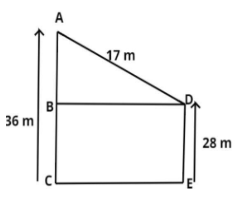Courses
Courses for Kids
Free study material
Free LIVE classes
MoreLIVE
Join Vedantu’s FREE Mastercalss

# The heights of two vertical poles are 36 m and 28 m. If the shortest distance between their tops is 17 m, find how far they (in m) are apart.Verified
360.9k+ views
Hint: In this question first draw the diagram it will give us a clear picture of what we have to find out, later on use the concept of Pythagoras Theorem to find out the solution of the question.The pictorial representation of the given problem is shown above.
Let AC and DE are two vertical poles of length 36 and 28 meter respectively.
It is given that the shortest distance between their tops is 17 meter.
$\Rightarrow AD = 17$ Meter (see figure).
Now we have to find out how far the vertical poles are apart.
Therefore we have to find out the value of BD (see figure)
Now in triangle ABD apply Pythagoras Theorem we have,
${\left( {{\text{Hypotenuse}}} \right)^2} = {\left( {{\text{Base}}} \right)^2} + {\left( {{\text{Perpendicular}}} \right)^2}$
$\Rightarrow {\left( {AD} \right)^2} = {\left( {BD} \right)^2} + {\left( {AB} \right)^2}$…………….. (1)
So, first calculate the distance AB.
As we see from figure AB is equal to the difference of the vertical poles length.
$\Rightarrow AB = AC - DE \\ \Rightarrow AB = 36 - 28 \\$
$\Rightarrow AB = 8$ meter.
Now from equation (1) we have
$\Rightarrow {\left( {17} \right)^2} = {\left( {BD} \right)^2} + {\left( 8 \right)^2} \\ \Rightarrow {\left( {BD} \right)^2} = 289 - 64 = 225 \\$
$\Rightarrow BD = \sqrt {225} = 15$ Meter.
So, the vertical poles are 15 meter apart.
So, this is the required answer.

Note: In such types of questions first draw the pictorial representation of the given problem as above, then first calculate the value of AB (see figure) by simply subtracting the length of vertical poles then apply the property of Pythagoras Theorem which is stated above and simplify, we will get the required distance between the poles (i.e. how far the vertical poles are apart).
Last updated date: 22nd Sep 2023
Total views: 360.9k
Views today: 3.60k